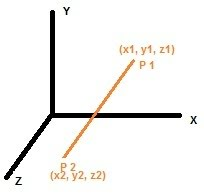### Distance between two points(3 dimension) calculation

Distance between two Points having 3 dimensional Cartesian coordinates, calculator calculates the distance between two given points co-ordinates (x1 , y1 , z1) and (x2 , y2 , z2)
Distance Formula : d = √ (x2 – x1)2 + (y2 - y1)2 + (z2 - z1)2X1: Y1: Z1: X2: Y2: Z2:

 Distance:

The distance between two points in 3D (three dimension) space is the square root of the sum of the squares of the differences between corresponding coordinates. Suppose two given point are (2,2,6) and (8,6,9), so we can find the differences between corresponding coordinates as follows: $$x = (x_{\,2} - x_{\,1}) , y = (y_{\,2} - y_{\,1}) \text{ and z }= (z_{\,2} - z_{\,1})$$ $$x = (8 - 2) , y = (6 - 2) \text{ and z }= (9 - 6)$$ $$x = 6 , y = 4 \text{ and z }= 3$$ $$\text{ now apply the formula for distance (between given two points in 3D) as follows: }$$ $$\sqrt {x^{2} + y^{2} + z^{2}}$$ $$\text{ therefore distance = } \sqrt {6^{2} + 4^{2} + 3^{2}}$$ $$= \sqrt {36 + 16 + 9}$$ $$= \sqrt {61}$$